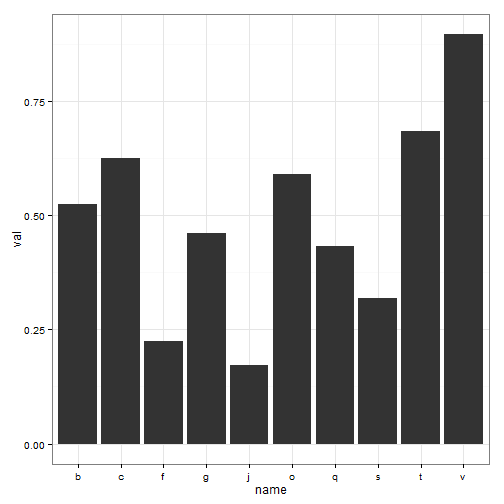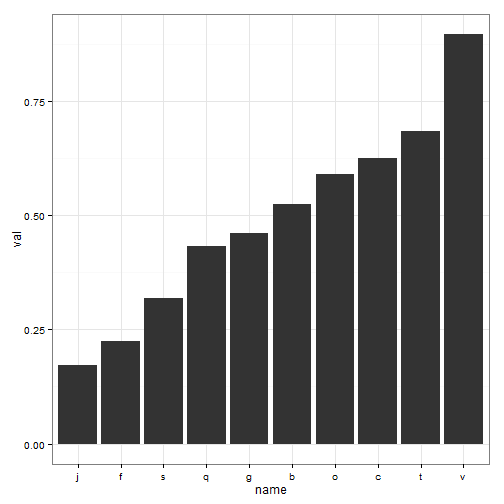# How to plot factors in a specified order in ggplot

A question of how to plot your data (in ggplot) in a desired order often comes up. Here's a quick demonstration of the trick you need to use to convince R and ggplot to do it.

For this exampe, we're assuming that you're trying to plot some factor variable on $$x$$ axis and $$y$$ axis holds some numeric values.

set.seed(357)
x <- data.frame(name = as.factor(sample(letters, 10)), val = runif(10))
x

##    name    val
## 1     c 0.6246
## 2     b 0.5246
## 3     g 0.4602
## 4     f 0.2255
## 5     o 0.5898
## 6     j 0.1717
## 7     s 0.3195
## 8     v 0.8953
## 9     t 0.6831
## 10    q 0.4317

str(x)

## 'data.frame':    10 obs. of  2 variables:
##  $name: Factor w/ 10 levels "b","c","f","g",..: 2 1 4 3 6 5 8 10 9 7 ##$ val : num  0.625 0.525 0.46 0.225 0.59 ...


Variable name is a factor, and the factor levels in it are ordered, by default, according to alphabet.

x$name  ##  c b g f o j s v t q ## Levels: b c f g j o q s t v  We might naively hope to arrange the data according to some criterion, let's say ascending order of val. x <- x[order(x$val), ]


and hope ggplot will read our mind. But we would be wrong, as demonstrated below.

library(ggplot2)
ggplot(x, aes(x = name, y = val)) + theme_bw() + geom_bar(stat = "identity")What we would like is for R to respect the order in data.frame. For that to happen, we need to change the order of factor levels by specifying the order explicitly.

x$name <- factor(x$name, levels = x$name[order(x$val)])
x\$name  # notice the changed order of factor levels

##   j f s q g b o c t v
## Levels: j f s q g b o c t v


ggplot will now understand in which order to plot name on $$x$$ axis.

ggplot(x, aes(x = name, y = val)) + theme_bw() + geom_bar(stat = "identity")